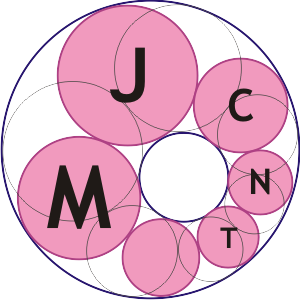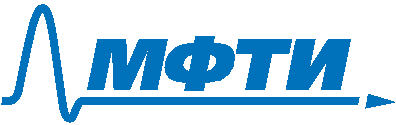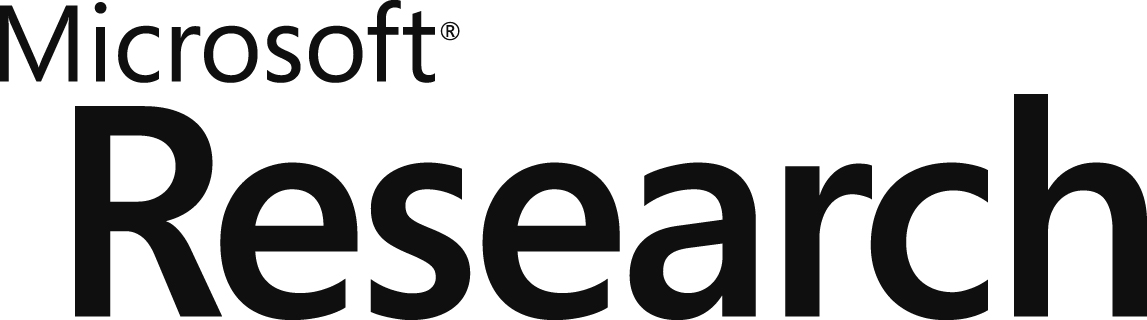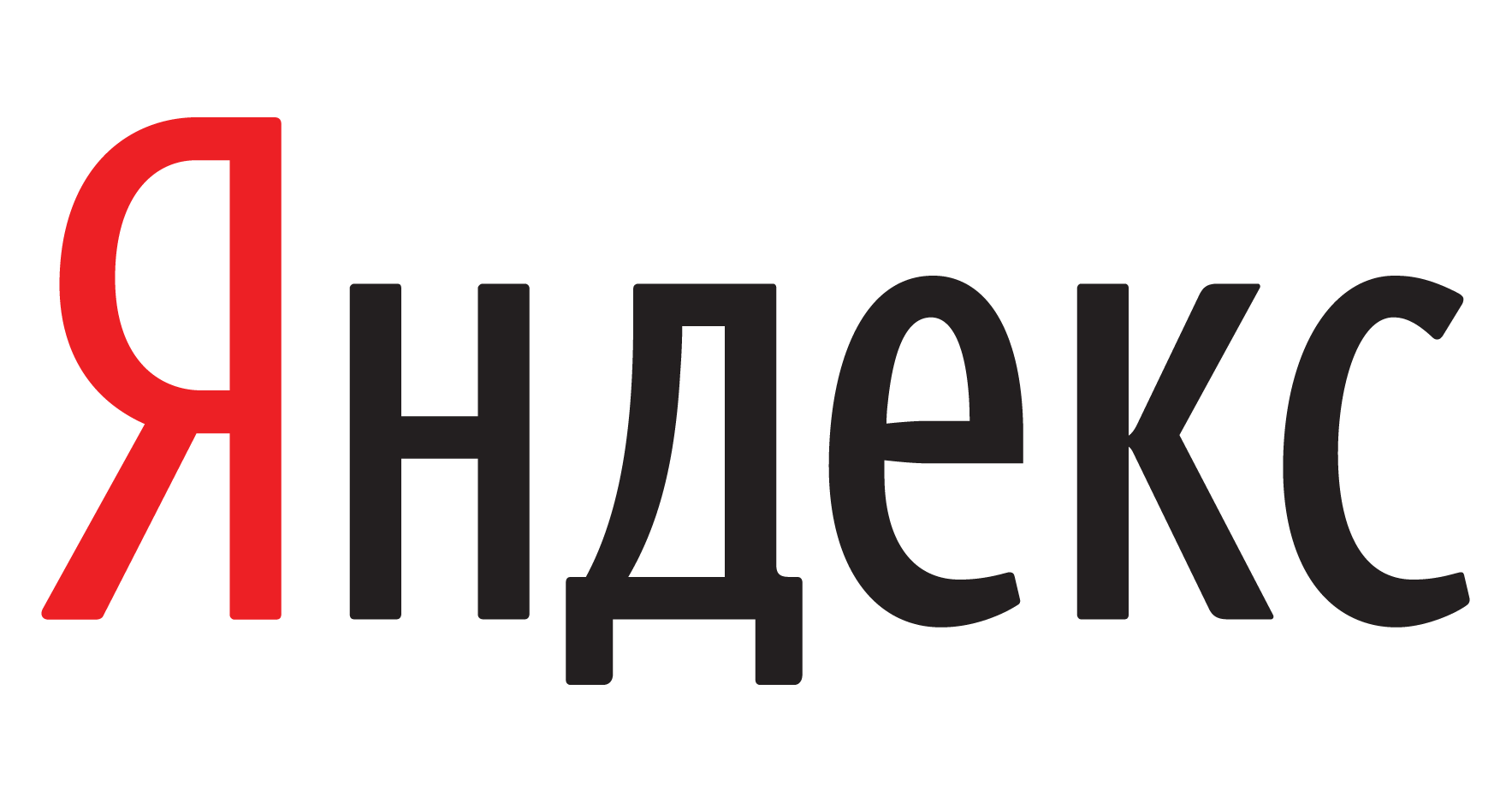Moscow Journal
of
Combinatorics and Number Theory
Main
Topics
Editorial board
Instructions for authors
Published volumes
Publisher
Call for papers
Subscription
Events
Authorized access

The journal publishes original research papers on topics in combinatorics and number theory; these are they:

#### Combinatorics

• Algebraic combinatorics
• Analytic combinatorics
• Coding theory
• Combinatorial, discrete and computational geometry
• Combinatorics on words
• Enumerative combinatorics
• Extremal combinatorics
• Geometric combinatorics
• Graph and hypergraph theory
• Partition theory
• Probabilistic combinatorics
• Topological combinatorics
• #### Number Theory

• Analytic number theory
• Applied number theory
• Combinatorial number theory
• Continued fractions
• Diophantine approximations
• Dynamical systems in number theory
• Elementary number theory
• Ergodic number theory
• Geometry of numbers
• Transcendence theory
• Uniform distribution of sequences
ISSN 2220-5438